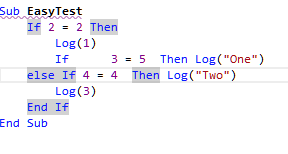# Android QuestionConditional operator logic

#### Genricke

##### Member
Hi !
Simple example: compiles and runs - no problem !

B4X:
``````Sub EasyTest
If 2 = 2 Then
Log(1)
If      3 = 5  Then Log("One")
else If 4 = 4  Then Log("Two")
Log(3)
End If
End Sub``````

Waiting: 1 -> Two -> 3
Reality: 1
Why ?! Thanks !Last edited:

#### Erel

##### B4X founder
Staff member
Longtime User
The IDE marks the condition block:I think that it explains the flow.

Correct code to get the result you expect:

B4X:
``````Sub EasyTest
If 2 = 2 Then
Log(1)
If 3 = 5 Then
Log("One")
Else If 4 = 4  Then
Log("Two")
End If
Log(3)
End If
End Sub``````

•MikeSW17 and emexes

Replies
3
Views
821
Android Question Bit.And operation
Replies
3
Views
2K
Replies
1
Views
4K